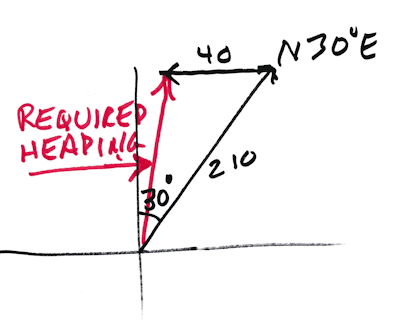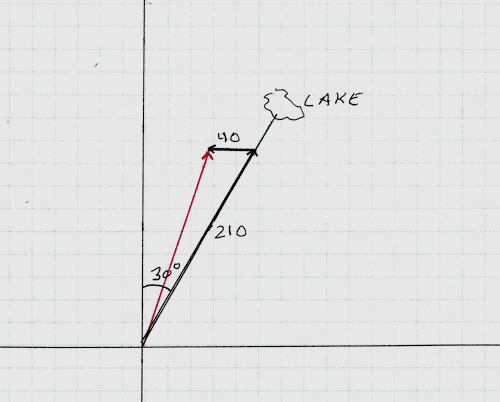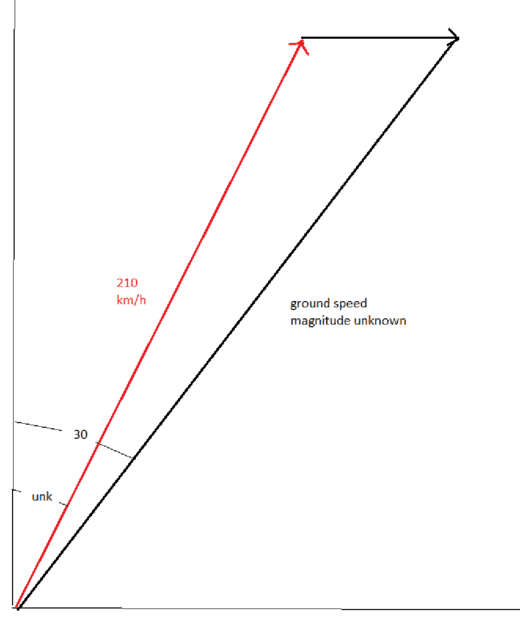# Is it possible to solve relative velocity problems without sine law?

Homework Statement:
A bush pilot wants to fly her plane to a lake that is 250.0 km [N30°E] from her starting point. The plane has an air speed of 210. km/h, and a wind blowing from the west at 40.0 km/h.

(a) In what direction should she head the plane to fly directly to the lake?

(b) If she uses the heading determined in (a), what will be her velocity relative to the ground?
Relevant Equations:
VPE = VPA + VAG
where VPE = Velocity of the plane relative to the ground
VPA = Velocity of the plane relative to the air
VAG = Velocity of the plane relative to the ground
I was able to solve this question successfully by utilizing the sine and cosine law however my instructor said I was only allowed to utilize the vector component method, I am unsure how to complete this question using the vector component method as we have two unknowns(those being the angle of the plane's velocity relative to the air and the magnitude of the velocity of the plane relative to the ground)

My closest attempt was when I utilized variables to attempt to solve for the magnitude of VPE which would allow me to rearrange the following equation:
VPE = x[N30E]
VPA = 210[unknown angle]
VAG = 40[E]

VPE = VPA - VAG
VPA = VPE - VAG -- we use this formula as we have the magnitude of VPA

This would allow me to obtain the direction she should head as well as the velocity relative to the ground

VPAx = xsin30 - 40
VPEy = xcos30

x = the magnitude of the velocity of the plane relative to the ground

The issue arises when I attempt to rearrange the Pythagorean equation which is as follows:

210^2 = (xsin30 - 40)^2-(xcos3o)^2

Note: 210 ^2 = magnitude of the plane relative to the air

When I attempt to rearrange the equation I am left with a quadratic equation whose roots don't have any significance and will not provide the correct value when inputted into the prior equations

All this has led me to believe that the only way to solve these questions is to utilize the sine and cosine laws

Any help is appreciated

Gold Member
2022 Award
You are making this way harder than it is. Just draw the vector components and you'll end up w/ a point that shows you the heading from the origin.

The trick is to ignore the distance to the target and focus on the DIRECTION to the target, then draw vector components of 210 and 40. The point at the end of those two vectors will give you the heading. You only need simple trig, not the sine law 'cause you'll be dealing w/ a couple of right triangles.

Last edited:
•berkeman and topsquark
You are making this way harder than it is. Just draw the vector components and you'll end up w/ a point that shows you the heading from the origin.

The trick is to ignore the distance to the target and focus on the DIRECTION to the target, then draw vector components of 210 and 40. The point at the end of those two vectors will give you the heading. You only need simple trig, not the sine law 'cause you'll be dealing w/ a couple of right triangles.
This would work provided we had the direction of 210, however, the question only specifies the magnitude of the velocity of the plane relative to the air(210km/h), if I attempt to draw the vectors with the directions given(direction of VPE and VAG), I end up with a triangle that is not a right triangle

Gold Member
2022 Award
This would work provided we had the direction of 210
What exactly do you think N30degreesE means?
Homework Statement:: A bush pilot wants to fly her plane to a lake that is 250.0 km [N30°E]

•malawi_glenn
Staff Emeritus
Homework Helper
The issue arises when I attempt to rearrange the Pythagorean equation which is as follows:

210^2 = (xsin30 - 40)^2-(xcos3o)^2
This should be ##210^2 = (x \sin 30^\circ - 40)^2 + (x \cos 30^\circ)^2##.

A more direct approach would be to write ##v_{{\rm PA},x} = v_{\rm PA} \cos\theta## and ##v_{{\rm PA},y} = v_{PA} \sin\theta##, and use the fact that ##v_{{\rm PE},x}/v_{{\rm PE},y} = \tan 30^\circ## so that the unknown speed of the plane relative to the ground cancels out.

Staff Emeritus
Homework Helper
What exactly do you think N30degreesE means?
That's the direction of ##\vec v_{\rm PE}##, not ##\vec v_{\rm PA}##.

Gold Member
2022 Award
That's the direction of ##\vec v_{\rm PE}##, not ##\vec v_{\rm PA}##.
I don't care what you label it, it is the direction of the airplane if there is no wind. Then you add in a vector for the wind. It's all trivial.

Homework Helper
Gold Member
Relevant Equations:: VPE = VPA + VAG
where VPE = Velocity of the plane relative to the ground
VPA = Velocity of the plane relative to the air
VAG = Velocity of the plane relative to the ground
This is confusing because there are two symbols for "Velocity of the plane relative to the ground"
The relevant velocities should be
##\mathbf{V}_{\text{PG}}## = velocity of Plane relative to the Ground
##\mathbf{V}_{\text{PA}}## = velocity of Plane relative to the Air
##\mathbf{V}_{\text{AG}}## = velocity of Air relative to the Ground
in which case the relevant equation is $$\mathbf{V}_{\text{PG}}=\mathbf{V}_{\text{PA}}+\mathbf{V}_{\text{AG}}.$$

•vela
Gold Member
2022 Award
I am absolutely flummoxed as to why you are all making a totally trivial vector addition problem into such a mish-mash of labels and stuff. To get part A, you have 3 numbers to deal with, 210, 30 and 40 and you just have to draw the vectors. It's like this. Here is what I said in post #2, but with a graphic.

Skip all the labels, just do the math (simple trig w/ right triangles).

I leave part B as another trivial exercise for the OP.•malawi_glenn
Staff Emeritus
Homework Helper
I am absolutely flummoxed as to why you are all making a totally trivial vector addition problem into such a mish-mash of labels and stuff. To get part A, you have 3 numbers to deal with, 210, 30 and 40 and you just have to draw the vectors. It's like this. Here is what I said in post #2, but with a graphic.

Skip all the labels, just do the math (simple trig w/ right triangles).

I leave part B as another trivial exercise for the OP.
View attachment 315327
And now reread the problem statement and recognize that your trivial solution is completely wrong.

willem2
Using the sine rule is using the vector component method. You are using a coordinate system with directions, parallel to and perpendicular to the desired direction of travel. If you set the perpendicular component to 0 you get the same equation as what the sine rule gives you.
If you use an angle φ to the left of the goal, this will produce a perpendicular velocity of 210 sin(φ) to the left, and the wind will produce a velocity of 40 sin(60 degrees) to the right. You only want a velocity in the direction of the goal, so the sum of those velocities will have to be 0.

•Lnewqban
Gold Member
2022 Award
And now reread the problem statement and recognize that your trivial solution is completely wrong.
I await your solution with baited breath and will be most interested in seeing how your answer differs from mine (hint ... it won't).

willem2
I am absolutely flummoxed as to why you are all making a totally trivial vector addition problem into such a mish-mash of labels and stuff. To get part A, you have 3 numbers to deal with, 210, 30 and 40 and you just have to draw the vectors. It's like this. Here is what I said in post #2, but with a graphic.

Skip all the labels, just do the math (simple trig w/ right triangles).

I leave part B as another trivial exercise for the OP.
View attachment 315327
The problem here is that the length of the vector you've drawn in the direction of the destination, is not equal to 210, The length of the red vector is equal to 210, but its direction is unknown.

Gold Member
2022 Award
The problem here is that the length of the vector you've drawn in the direction of the destination, is not equal to 210, The length of the red vector is equal to 210, but its direction is unknown.
As I have clearly stated, the 210 vector is correct as shown if there is no wind. But since there IS wind, the 40 vector is introduced to compensate for it.

It is true that the plane has an airspeed of 210mph and thus even though it will travel along the PATH of the 210 vector, since the wind has been compensated for, it will not reach the end of the 210 arrow after exactly 1 hour. That does not change the correctness of my solution. The plane will travel along the 210 vector througout but it will be POINTED throughout along the red vector. Thus the HEADING is the red vector and the actual path is the 210 vector, which is what gets the plane to the correct destination by compensating for the wind.

I say again, this is a trivial vector addition problem.

Homework Helper
Gold Member
With no wind, the airplane would point its nose in the direction of the lake and will arrive there in a time of 250/210 hour.
With wind from the West, there is a component of that wind pushing the airplane from its tail; therefore, it should arrive to the lake sooner as its ground speed should increase some.
Think of a square tailwind coming from the SSW instead.

Staff Emeritus
Homework Helper
I await your solution with baited breath and will be most interested in seeing how your answer differs from mine (hint ... it won't).
Consider that everybody else who has contributed to this thread, including the OP, disagrees with you and that several people have pointed out your mistake explicitly. Then entertain the notion that you are, in fact, the one who might be wrong, as impossible as that may seem to be. Then reread the problem statement and the responses in this thread carefully.

Gold Member
2022 Award
Consider that everybody else who has contributed to this thread, including the OP, disagrees with you and that several people have pointed out your mistake explicitly. Then entertain the notion that you are, in fact, the one who might be wrong, as impossible as that may seem to be. Then reread the problem statement and the responses in this thread carefully.
See my post #15.

Staff Emeritus
Homework Helper
See my post #15.
Consider that yet another poster has pointed out a problem with your analysis.

Gold Member
2022 Award
With no wind, the airplane would point its nose in the direction of the lake and will arrive there in a time of 250/210 hour.
With wind from the West, there is a component of that wind pushing the airplane from its tail; therefore, it should arrive to the lake sooner as its ground speed should increase some.
Think of a square tailwind coming from the SSW instead.
Yep, you are right. That does nothing to change my analysis of the required heading, which is the red vector. My initial drawing was a quick sketch to show the form of the solution but was not done to scale. Here's one to scale and since the required heading remains to the East of North, you are correct that the wind from the West is partially a tailwind. Had the wind been very much over 100mph then the required heading would have been to the West of North and the West wind would have had headwind component.•Lnewqban
Staff Emeritus
Homework Helper
That does nothing to change my analysis of the required heading
It's still wrong. I calculated the heading both ways, and I got two different answers.

Your analysis amounts to the claim that you can swap two sides of a scalene triangle without changing any of the angles.

Gold Member
2022 Award
It's still wrong.
Unless you can show me how it is not a simple vector addition problem, solved as I show, then we're going to have to just agree to disagree. We see this kind of problem in introductory vectors all the time, as I'm sure you know. Planes w/ crosswind, boats moving across the current and so forth. They're all just simple vector addition problems.

Homework Helper
Gold Member
For whatever it's worth, one can find on the web calculators that will give the answer to this problem given the parameters and check one's answers regardless of the method one followed.

Homework Helper
See my post #15.
Let me try.

From the OP we have:
A bush pilot wants to fly her plane to a lake that is 250.0 km [N30°E] from her starting point. The plane has an air speed of 210. km/h, and a wind blowing from the west at 40.0 km/h.

(a) In what direction should she head the plane to fly directly to the lake?

In your drawing, we have the plane's air speed (210 km/h) depicted on a course over the ground 30 degrees east of due north.

That means that the drawing is all screwed up.

The vector of unknown magnitude (ground speed) should be on the known course of 30 degrees.

The vector of magnitude 210 km/h (air speed) should be on an unknown heading. That is what we are trying to determine.

The vector of magnitude 40 km/h is drawn correctly.

But we need to position the arrows and vectors properly for their new interpretations. Like so:Edit: Updated with the note from @vela that the wind speed is eastward, not westward.

Still not identical to @phinds because the angle labels and the speed labels are on different vectors.

It is not proper to put an air speed on a ground velocity vector.

Last edited:
Staff Emeritus
Homework Helper
The vector of magnitude 40 km/h is drawn correctly.
The problem statement said the wind is from the west, so it's blowing to the east.

•jbriggs444
•